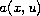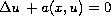Electron. J. Diff. Equ., Vol. 2010(2010), No. 37, pp. 1-5.

### An inverse boundary-value problem for semilinear elliptic equations Ziqi Sun

Abstract:
We show that in dimension two or greater, a certain equivalence class of the scalar coefficientof the semilinear elliptic equationis uniquely determined by the Dirichlet to Neumann map of the equation on a bounded domain with smooth boundary. We also show that the coefficientcan be determined by the Dirichlet to Neumann map under some additional hypotheses.

Submitted January 31, 2010. Published March 14, 2010.
Math Subject Classifications: 35R30.
Key Words: Inverse Problem; Dirichlet to Neumann map.

Show me the PDF file (175 KB), TEX file, and other files for this article.Ziqi Sun Department of Mathematics and Statistics Wichita State University, Wichita, KS 67260-0033, USA email: ziqi.sun@wichita.edu